【GiantPandaCV导语】重新思考可变形卷积。逐行代码解析，希望读者能一起完全理解DCN并明白DCN的好处，同时也清楚DCN的缺点在哪里。最后对什么时候可以选择DCN有一个较好的认识。添加了注释的代码链接：https://github.com/BBuf/pytorch-deform-conv-v2-explain

# 0x01. DCN V1原理回顾¶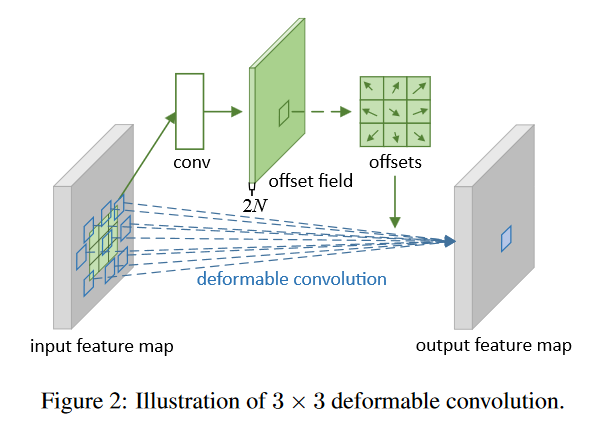• 原始图片数据（维度是$b*h*w*c$），记为U。经过一个普通卷积，填充方式为same，对应的输出结果维度是$(b*h*w*2c)$，记作V。V是原始图像数据中每个像素的偏移量（因为有$x$$y$两个方向，所以是$2c$）。
• 将U中图片的像素索引值与V相加，得到偏移后的position（即在原始图片U中的坐标值），需要将position值限定为图片大小以内。position的大小为（$b*h*w*2c$)，但position只是一个坐标值，而且还是float类型的，我们需要这些float类型的坐标值获取像素。
• 举个例子，我们取一个坐标值$(a,b)$，将其转换为四个整数floor(a), ceil(a), floor(b), ceil(b)，将这四个整数进行整合，得到四对坐标（floor(a),floor(b)), ((floor(a),ceil(b)), ((ceil(a),floor(b)), ((ceil(a),ceil(b))。这四对坐标每个坐标都对应U中的一个像素值，而我们需要得到(a,b)的像素值，这里采用双线性差值的方式计算（一方面是因为获得的像素更精确，另外一方面是因为可以进行反向传播）。
• 在得到position的所有像素后，即得到了一个新图片M，将这个新图片M作为输入数据输入到别的层中，如普通卷积。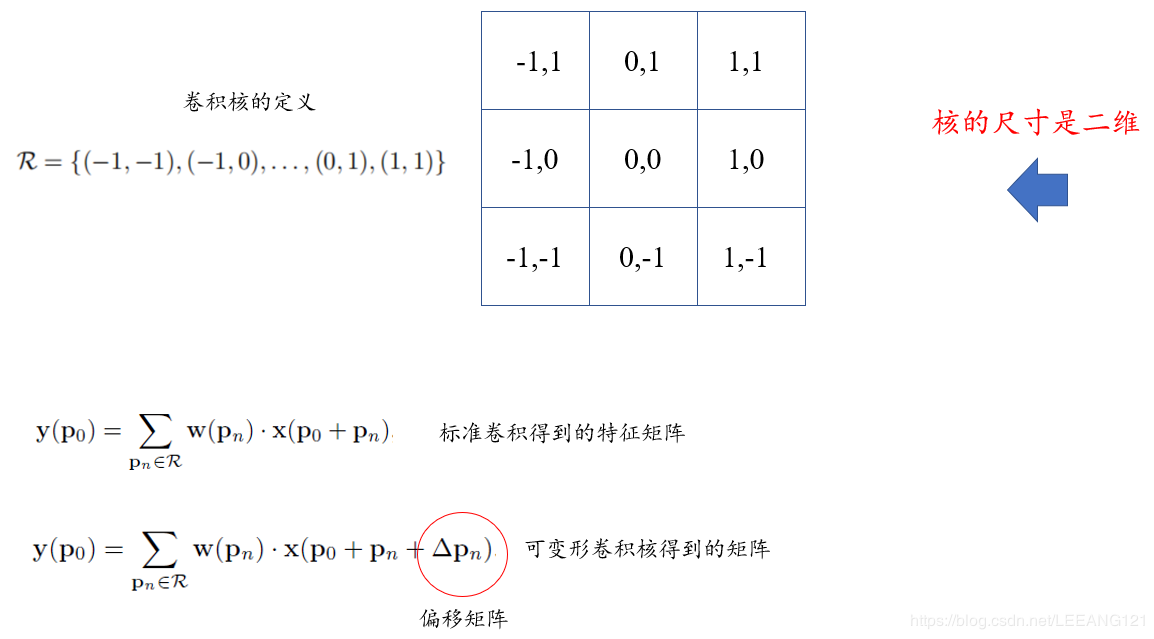# 0x02. DCNV2?¶

$y(p)=\sum_{k=1}^Kw_k * x(p + p_k+\Delta_{p_k})$

CNN要学习的就是这个偏移量$\Delta{p_k}$

$y(p)=\sum_{k=1}^Kw_k * x(p + p_k+\Delta_{p_k}) * \Delta m_k$

# 0x03. 代码解析¶

## 0x03.1 类的构造¶

# inc表示输入通道数
# outc 表示输出通道数
# kernel_size表示卷积核尺寸
# stride 卷积核滑动步长
# bias 偏置
# modulation DCNV1还是DCNV2的开关
def __init__(self, inc, outc, kernel_size=3, padding=1, stride=1, bias=None, modulation=False):
"""
Args:
modulation (bool, optional): If True, Modulated Defomable Convolution (Deformable ConvNets v2).
"""
super(DeformConv2d, self).__init__()
self.kernel_size = kernel_size
self.stride = stride
# 普通的卷积层，即获得了偏移量之后的特征图再接一个普通卷积
self.conv = nn.Conv2d(inc, outc, kernel_size=kernel_size, stride=kernel_size, bias=bias)
# 获得偏移量，卷积核的通道数应该为2xkernel_sizexkernel_size
self.p_conv = nn.Conv2d(inc, 2*kernel_size*kernel_size, kernel_size=3, padding=1, stride=stride)
# 偏移量初始化为0
nn.init.constant_(self.p_conv.weight, 0)
# 注册module反向传播的hook函数, 可以查看当前层参数的梯度
self.p_conv.register_backward_hook(self._set_lr)
# 将modulation赋值给当前类
self.modulation = modulation
if modulation:
# 如果是DCN V2，还多了一个权重参数，用m_conv来表示
self.m_conv = nn.Conv2d(inc, kernel_size*kernel_size, kernel_size=3, padding=1, stride=stride)
nn.init.constant_(self.m_conv.weight, 0)
# 注册module反向传播的hook函数, 可以查看当前层参数的梯度
self.m_conv.register_backward_hook(self._set_lr)

# 静态方法 类或实例均可调用，这函数的结合hook可以输出你想要的Variable的梯度
@staticmethod


## 0x03.2 _get_p函数¶实际上这个函数就需要分别求取红色块的位置，蓝色块的位置，然后再加上网络学习到的offsets获得最终的偏移坐标。

def _get_p(self, offset, dtype):
# N = 18 / 2 = 9
# h = 32
# w = 32
N, h, w = offset.size(1)//2, offset.size(2), offset.size(3)

# (1, 2N, 1, 1)
p_n = self._get_p_n(N, dtype)
# (1, 2N, h, w)
p_0 = self._get_p_0(h, w, N, dtype)
# 卷积坐标加上之前学习出的offset后就是论文提出的公式(2)也就是加上了偏置后的卷积操作。
# 比如p(在N=0时)p_0就是中心坐标，而p_n=(-1,-1)，所以此时的p就是卷积核中心坐标加上
# (-1,-1)(即红色块左上方的块)再加上offset。同理可得N=1,N=2...分别代表了一个卷积核
# 上各个元素。
p = p_0 + p_n + offset
return p


def _get_p_0(self, h, w, N, dtype):
# 设w = 7, h = 5, stride = 1
# 有p_0_x = tensor([[1, 1, 1, 1, 1, 1, 1],
# [2, 2, 2, 2, 2, 2, 2],
# [3, 3, 3, 3, 3, 3, 3],
# [4, 4, 4, 4, 4, 4, 4],
# [5, 5, 5, 5, 5, 5, 5]])
# p_0_x.shape = [5, 7]
# p_0_y = tensor([[1, 2, 3, 4, 5, 6, 7],
# [1, 2, 3, 4, 5, 6, 7],
# [1, 2, 3, 4, 5, 6, 7],
# [1, 2, 3, 4, 5, 6, 7],
# [1, 2, 3, 4, 5, 6, 7]])
# p_0_y.shape = [5, 7]
p_0_x, p_0_y = torch.meshgrid(
torch.arange(1, h*self.stride+1, self.stride),
torch.arange(1, w*self.stride+1, self.stride))
# p_0_x的shape为torch.Size([1, 9, 5, 7])
p_0_x = torch.flatten(p_0_x).view(1, 1, h, w).repeat(1, N, 1, 1)
# p_0_y的shape为torch.Size([1, 9, 5, 7])
p_0_y = torch.flatten(p_0_y).view(1, 1, h, w).repeat(1, N, 1, 1)
# p_0的shape为torch.Size([1, 18, 5, 7])
p_0 = torch.cat([p_0_x, p_0_y], 1).type(dtype)

return p_0


## 0x03.3 forward函数¶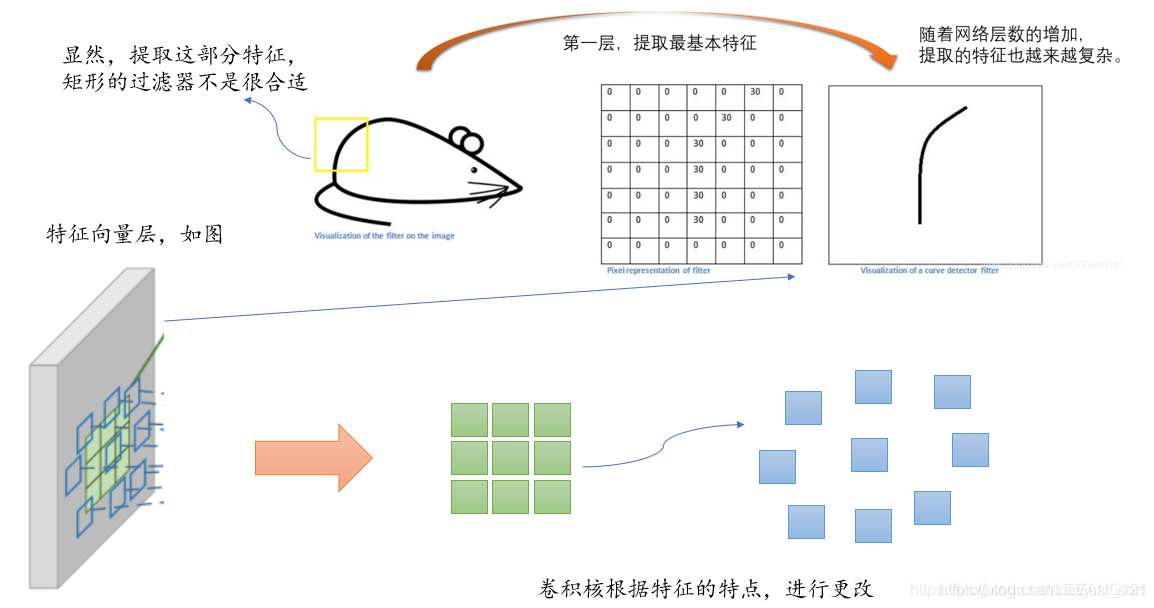这部分的详细代码解释如下：

# 前向传播函数
def forward(self, x):
# 获得输入特征图x的偏移量
# 假设输入特征图shape是[1,3,32,32]，然后卷积核是3x3，
# 输出通道数为32，那么offset的shape是[1,2*3*3,32]
offset = self.p_conv(x)
# 如果是DCN V2那么还需要获得输入特征图x偏移量的权重项
# 假设输入特征图shape是[1,3,32,32]，然后卷积核是3x3，
# 输出通道数为32，那么offset的权重shape是[1,3*3,32]
if self.modulation:
m = torch.sigmoid(self.m_conv(x))
# dtype = torch.float32
dtype = offset.data.type()
# 卷积核尺寸大小
ks = self.kernel_size
# N=2*3*3/2=3*3=9
N = offset.size(1) // 2

# p的shape为(b, 2N, h, w)
# 这个函数用来获取所有的卷积核偏移之后相对于原始特征图x的坐标（现在是浮点数）
p = self._get_p(offset, dtype)

# 我们学习出的量是float类型的，而像素坐标都是整数类型的，
# 所以我们还要用双线性插值的方法去推算相应的值
# 维度转换，现在p的维度为(b, h, w, 2N)
p = p.contiguous().permute(0, 2, 3, 1)
# floor是向下取整
q_lt = p.detach().floor()
# +1相当于原始坐标向上取整
q_rb = q_lt + 1
# 将q_lt即左上角坐标的值限制在图像范围内
q_lt = torch.cat([torch.clamp(q_lt[..., :N], 0, x.size(2)-1), torch.clamp(q_lt[..., N:], 0, x.size(3)-1)], dim=-1).long()
# 将q_rb即右下角坐标的值限制在图像范围内
q_rb = torch.cat([torch.clamp(q_rb[..., :N], 0, x.size(2)-1), torch.clamp(q_rb[..., N:], 0, x.size(3)-1)], dim=-1).long()
# 用q_lt的前半部分坐标q_lt_x和q_rb的后半部分q_rb_y组合成q_lb
q_lb = torch.cat([q_lt[..., :N], q_rb[..., N:]], dim=-1)
# 同理
q_rt = torch.cat([q_rb[..., :N], q_lt[..., N:]], dim=-1)

# 对p的坐标也要限制在图像范围内
p = torch.cat([torch.clamp(p[..., :N], 0, x.size(2)-1), torch.clamp(p[..., N:], 0, x.size(3)-1)], dim=-1)

# bilinear kernel (b, h, w, N)
# 双线性插值的4个系数
g_lt = (1 + (q_lt[..., :N].type_as(p) - p[..., :N])) * (1 + (q_lt[..., N:].type_as(p) - p[..., N:]))
g_rb = (1 - (q_rb[..., :N].type_as(p) - p[..., :N])) * (1 - (q_rb[..., N:].type_as(p) - p[..., N:]))
g_lb = (1 + (q_lb[..., :N].type_as(p) - p[..., :N])) * (1 - (q_lb[..., N:].type_as(p) - p[..., N:]))
g_rt = (1 - (q_rt[..., :N].type_as(p) - p[..., :N])) * (1 + (q_rt[..., N:].type_as(p) - p[..., N:]))

# (b, c, h, w, N)
# 现在只获取了坐标值，我们最终木的是获取相应坐标上的值，
# 这里我们通过self._get_x_q()获取相应值。
x_q_lt = self._get_x_q(x, q_lt, N)
x_q_rb = self._get_x_q(x, q_rb, N)
x_q_lb = self._get_x_q(x, q_lb, N)
x_q_rt = self._get_x_q(x, q_rt, N)

# (b, c, h, w, N)
# 双线性插值计算
x_offset = g_lt.unsqueeze(dim=1) * x_q_lt + \
g_rb.unsqueeze(dim=1) * x_q_rb + \
g_lb.unsqueeze(dim=1) * x_q_lb + \
g_rt.unsqueeze(dim=1) * x_q_rt

# modulation
if self.modulation:
m = m.contiguous().permute(0, 2, 3, 1)
m = m.unsqueeze(dim=1)
m = torch.cat([m for _ in range(x_offset.size(1))], dim=1)
x_offset *= m

# 在获取所有值后我们计算出x_offset，但是x_offset的size
# 是(b,c,h,w,N)，我们的目的是将最终的输出结果的size变
# 成和x一致即(b,c,h,w)，所以在最后用了一个reshape的操作。
# 这里ks=3
x_offset = self._reshape_x_offset(x_offset, ks)
out = self.conv(x_offset)

return out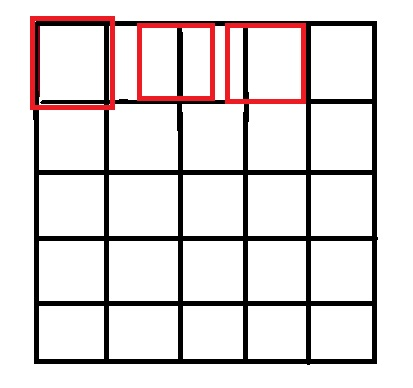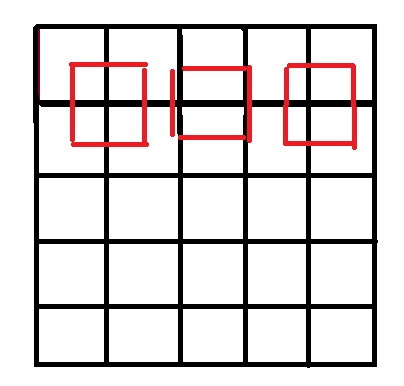int x=i*m/a;
int y=j*n/b;


int x=(i+0.5)*m/a-0.5;
int y=(j+0.5)*n/b-0.5;


https://github.com/BBuf/Image-processing-algorithm/blob/master/Image%20Interpolation/BilinearInterpolation.cpp

Mat BilinearInterpolation(Mat src, float sx, float sy) {
int row = src.rows;
int col = src.cols;
int channels = src.channels();
int dst_row = round(row * sx);
int dst_col = round(col * sy);
Mat dst(dst_row, dst_col, CV_8UC3);
for (int i = 0; i < dst_row; i++) {
int index_i = (i + 0.5) / sx - 0.5;
if (index_i < 0) index_i = 0;
if (index_i > row - 2) index_i = row - 2;
int i1 = floor(index_i);
int i2 = ceil(index_i);
float u = index_i - i1;
for (int j = 0; j < dst_col; j++) {
float index_j = (j + 0.5) / sy - 0.5;
if (index_j < 0) index_j = 0;
if (index_j > col - 2) index_j = col - 2;
int j1 = floor(index_j);
int j2 = ceil(index_j);
float v = index_j - j1;
for (int k = 0; k < 3; k++) {
dst.at<cv::Vec3b>(i, j)[k] = (1 - u)*(1 - v)*src.at<cv::Vec3b>(i1, j1)[k] +
(1 - u)*v*src.at<cv::Vec3b>(i1, j2)[k] + u*(1 - v)*src.at<cv::Vec3b>(i2, j1)[k] + u*v*src.at<cv::Vec3b>(i2, j2)[k];
}
}
}
return dst;
}


# 双线性插值的4个系数
g_lt = (1 + (q_lt[..., :N].type_as(p) - p[..., :N])) * (1 + (q_lt[..., N:].type_as(p) - p[..., N:]))
g_rb = (1 - (q_rb[..., :N].type_as(p) - p[..., :N])) * (1 - (q_rb[..., N:].type_as(p) - p[..., N:]))
g_lb = (1 + (q_lb[..., :N].type_as(p) - p[..., :N])) * (1 - (q_lb[..., N:].type_as(p) - p[..., N:]))
g_rt = (1 - (q_rt[..., :N].type_as(p) - p[..., :N])) * (1 + (q_rt[..., N:].type_as(p) - p[..., N:]))


## 0x03.4 _reshape_x_offset函数¶

def _reshape_x_offset(x_offset, ks):
# 函数首先获取了x_offset的所有size信息，然后以kernel_size为
# 单位进行reshape，因为N=kernel_size*kernel_size，所以我们
# 分两次进行reshape，第一次先把输入view成(b,c,h,ks*w,ks)，
# 第二次再view将size变成(b,c,h*ks,w*ks)

b, c, h, w, N = x_offset.size()
x_offset = torch.cat([x_offset[..., s:s+ks].contiguous().view(b, c, h, w*ks) for s in range(0, N, ks)], dim=-1)
x_offset = x_offset.contiguous().view(b, c, h*ks, w*ks)

return x_offset$y(p)=\sum_{k=1}^Kw_k * x(p + p_k+\Delta_{p_k})$